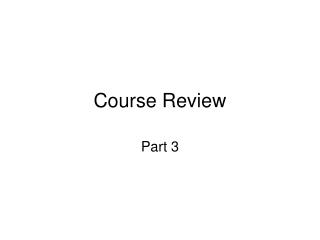DownloadDownload PresentationCourse Review

# Course Review

Télécharger la présentation## Course Review

- - - - - - - - - - - - - - - - - - - - - - - - - - - E N D - - - - - - - - - - - - - - - - - - - - - - - - - - -
##### Presentation Transcript

1. Course Review Part 3

2. Manual stability control • Manual servo control

3. 6.6 Reset windup • The condition where the integral action drives the controller output to one of its limits. • Usually because of • Poor configuration • Undersized (or oversized) valve • The controller cannot reach setpoint - offset. This offset causes the integral term to wind up • The controller may be slow in responding to a change in the controlled variable because of the dominant integral term

4. Reset Windup SP PV Output limit Controller Output

5. 7. Cascade Motivating Example • Valve may be nonlinear • Steam supply disturbances are fast, but can only be mitigated once they affect temperature • Steam dynamics may be complicated or even unstable Steam supply disturbances Temperature disturbances d2 d1 Desired Steam flow valve position Real World Gd2 Gd1 Actual Valve Position Temp Error in C Desired temp in C Steam flow Temp Gc1 Gv Gp2 Gp1 y + - Gm1

6. Cascade mitigates: • Nonlinearities in the valve • Fast disturbances in d2 • Dynamics in Gd2 • Instability or dynamics in Gp2 • Split the control problem into two parts: • Steam flow • Temperature control, given steam flow • If inner loop  < outer loop /5, then the inner loop can be ignored Steam supply disturbances Temperature disturbances d2 d1 Real World Gd2 Gd1 Desired Steam flow Temp Error in C Valve Position Desired temp in C Steam flow Temp Gc1 Gc2 Gv Gp2 Gp1 y + + - - Gm2 Gm1

7. 8. Feedforward • Feedback is mostly about the poles of the transfer function: long term behaviour and stability • Feedforward is about the zeros of the transfer function: short term dynamics • Feedback cannot affect the zeros of a transfer function. Feedforward can. • Can be very useful if there is a long time delay in Gd and Gp.

8. Change in Pressure d Gff Gd Feedforward Boiler steam drum level control: Process shows inverse response: confuses feedback control. RHP zero can be mitigated by feedforward. For perfect cancellation Boiler Feed Water flow Level • Zeros in Gd are modified by adding Gff * Gp. • Perfect feedforward is not possible if: • delay in Gp is greater than delay in Gd, (requires future values of disturbance) • Gp has RHP zeros (Gff would be unstable) • In such cases, use static feedforward, or leave out the unstable part. Gp y

9. 9. Routh Stability Criterion • Useful to find limiting values of Kc and I • Can only be used on polynomials • If characteristic equation contains exponentials, use a Pade approximation • Write characteristic equation as a polynomial: Make coefficient of highest power of s (an) positive If any coefficient is negative or zero, system is not stable 2. If all coefficients are positive, construct Routh array:

10. Routh Array will have n+1 rows Each row has one less column than the row above. Row n+1 will have only one column. The number of unstable poles is equal to the number of sign changes going down the first column of the array

11. Calculation

12. 10. Frequency Response

13. Amplitude Ratio and Phase Shift using Transfer Functions • Replace S with j in the transfer function: G(s) G(j) • Rationalize G: make it equal to a + jb, where a and b may be functions of  (G is now a complex number that is a function of ) • AR = |G| = sqrt(a2 + b2) •  = tan-1(b/a)

14. Amplitude Ratio and Phase Shift using Transfer Functions For systems in series: Gc Gv Gp Input Output Transfer functions multiply: G = Gc * Gv * Gp Amplitude ratios multiply and Phase angles add: AR = AR(Gc) * AR(Gv) * AR(Gp) = (Gc) + (Gv) + (Gp) Logarithms of Amplitude Ratios add: Log(AR) = log(AR(Gc)) + log(AR(Gv)) + log(AR(Gp))

15. 10.4 Bode Stability Criterion Output amplitude Input amplitude Input Output Controller Valve Process - c is the frequency at which the phase shift of the forward path = -180o If the AR at c < 1, then the system is stable. The “-” sign in the negative feedback gives another 180o At phase lag of 180o for the forward path, input = previous input*AR

16. AR = 1 10.4 Bode Stability Criterion, Gain and Phase Margin Gain Margin AR < 1 at c, so system is stable g c Phase Margin  = -180

17. 11. Multivariable Systems

18. Break

19. Feedback • Control of unstable system